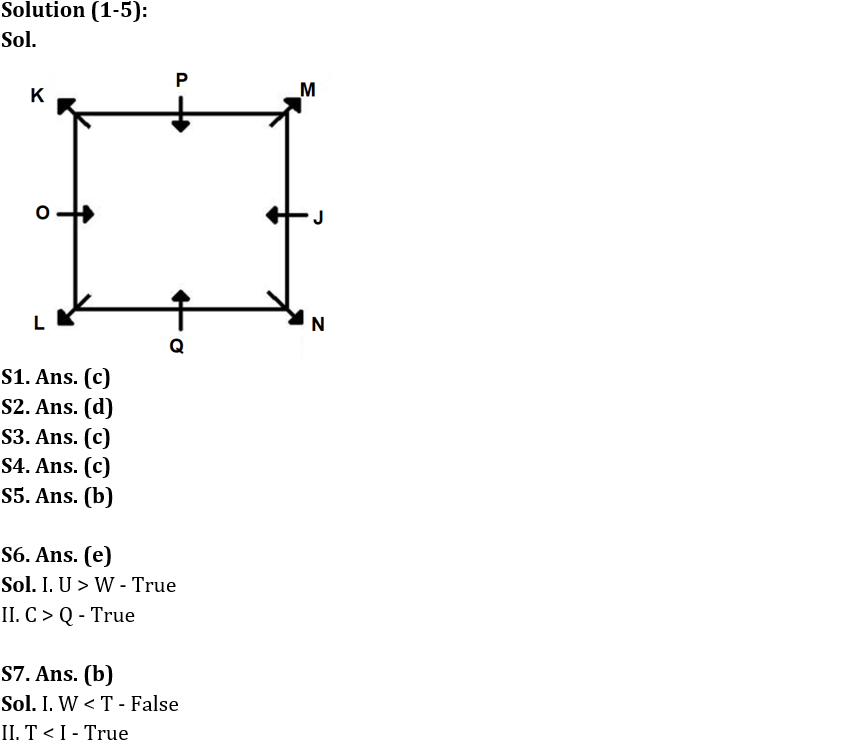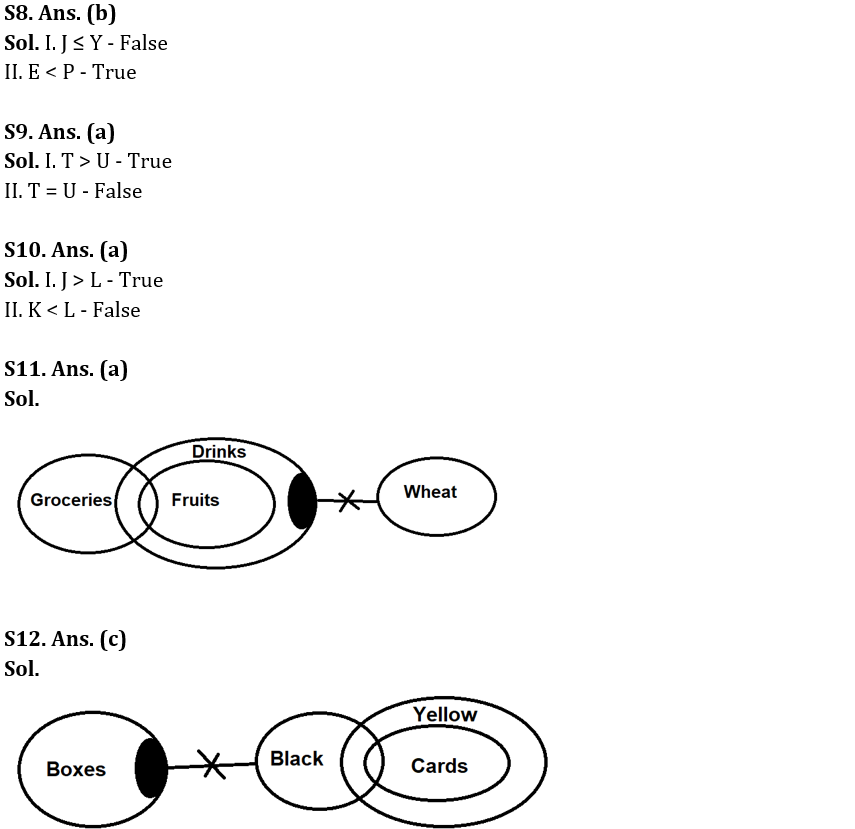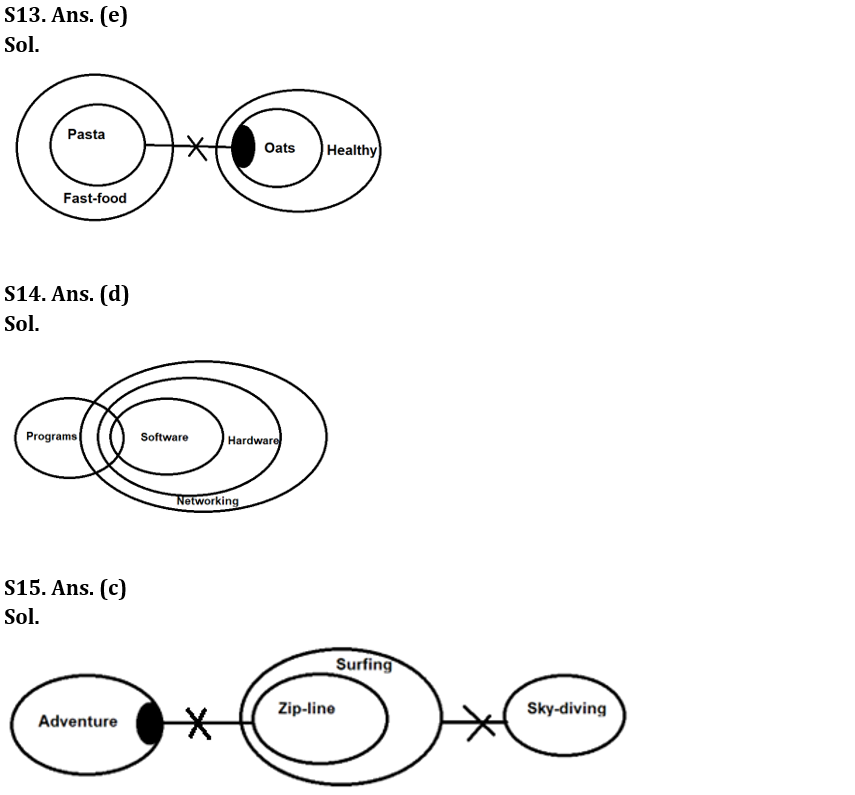Latest Banking jobs   »

# Reasoning Ability Quiz For IBPS RRB PO Prelims 2022- 20th June

Directions (1-5): Study the information carefully and answer the following questions.
Eight people i.e., J, K, L, M, N, O, P and Q sit around a square-shaped table. Four people sit at the four corners of the table and face outside the table. Four people sit at the middle of the four sides and face inside the table. Only one person sits between J and Q who sits at the middle of the side. K sits third to the left of Q. M sits second to the right of K. Two people sit between M and O. L sits second to the right of N.

Q1. Who sits third to the right of N?
(a) Q
(b) L
(c) O
(d) K
(e) None of these

Q2. Which of the following statements is true?
(a) Q faces M
(b) K sits second to left of J
(c) Two people sit between N and J
(d) O faces J
(e) None is true

Q3. Four of the following five are alike in a certain way and hence form a group. Find the one who does not belong to that group.
(a) Q
(b) P
(c) M
(d) J
(e) O

Q4. How many people sit between P and M when counted from left of P?
(a) Three
(b) One
(c) None
(d) Four
(e) Two

Q5. Who sits second to the left of the one who sits immediate left of J?
(a) N
(b) M
(c) P
(d) K
(e) None of these

Directions (6-10): In the following questions assuming the given statement to be true, find which of the conclusion(s) among given conclusions is/are definitely true and then give your answers accordingly.

Q6. Statements: Q ≤ W ≤ G, C > U > G > O > V
Conclusions: I. U > W
II. C > Q
(a) Only I is true
(b) Only II is true
(c) Either I or II is true
(d) Neither I nor II is true
(e) Both I and II are true

Q7. Statements: D > H > T, W ≥ P ≥ H < I
Conclusions: I. W < T
II. T < I
(a) Only I is true
(b) Only II is true
(c) Either I or II is true
(d) Neither I nor II is true
(e) Both I and II are true

Q8. Statements: U < P < Y, J ≥ F > E, S = U ≥ E
Conclusions: I. J ≤ Y
II. E < P
(a) Only I is true
(b) Only II is true
(c) Either I or II is true
(d) Neither I nor II is true
(e) Both I and II are true

Q9. Statements: T > A ≥ G, G = U > E, T ≥ F
Conclusions: I. T > U
II. T = U
(a) Only I is true
(b) Only II is true
(c) Either I or II is true
(d) Neither I nor II is true
(e) Both I and II are true

Q10. Statements: J > A ≥ K, K = U > E, J ≥ F > L
Conclusions: I. J > L
II. K < L
(a) Only I is true
(b) Only II is true
(c) Either I or II is true
(d) Neither I nor II is true
(e) Both I and II are true

Directions (11-15): In the question below, three statements are given followed by the conclusions. You have to take the given statements to be true even if they seem to be at variance with commonly known facts. Read all the conclusions and then decide which of the given conclusions logically follows from the given statements disregarding commonly known facts.

Q11. Statements: Only a few Groceries are Fruits. All Fruits are Drinks. Some Drinks are not Wheat.
Conclusions: I. All Groceries can be Drinks
II. Some Fruits are not Wheat
(a) Only conclusion I follows
(b) Only conclusion II follows
(c) Either conclusion I or II follows
(d) Both conclusions I and II follow
(e) Neither conclusion I nor II follows

Q12. Statements: Some Boxes are not Black. Only a few Black are Cards. All Cards are Yellow.
Conclusions: I. Some Boxes are Yellow
II. No Boxes are Yellow
(a) Only conclusion I follows
(b) Only conclusion II follows
(c) Either conclusion I or II follows
(d) Both conclusions I and II follow
(e) Neither conclusion I nor II follows

Q13. Statements: All Pasta are Fast-food. Some Oats are not Pasta. All Oats are Healthy.
Conclusions: I. No Pastas are Oats
II. Some Healthy are not Fast-foods
(a) Only conclusion I follows
(b) Only conclusion II follows
(c) Either conclusion I or II follows
(d) Both conclusions I and II follow
(e) Neither conclusion I nor II follows

Q14. Statements: Only a few Programs are Software. All Software are Hardware. All Hardware are Networking.
Conclusions: I. All Software are Networking
II. All Programs can be Networking
(a) Only conclusion I follows
(b) Only conclusion II follows
(c) Either conclusion I or II follows
(d) Both conclusions I and II follow
(e) Neither conclusion I nor II follows

Q15. Statements: Some Adventure is not Zip-line. All Zip-line is Surfing. No Surfing is Sky-diving.
Conclusions: I. Some Adventure is Sky-diving
(a) Only conclusion I follows
(b) Only conclusion II follows
(c) Either conclusion I or II follows
(d) Both conclusions I and II follow
(e) Neither conclusion I nor II follows

Solutions#### Congratulations!